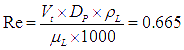# Vertical separator sizing

Vertical separators are commonly used in the oil and gas industry to separate a mixture of liquids and gases. These separators use gravity to separate the fluids based on their densities, with the heavier fluids settling to the bottom of the vessel and the lighter fluids rising to the top. In this post, we will explore the theory behind separator sizing and provide a step-by-step guide for vertical separator sizing calculation to help engineers and designers accurately size vertical separators for their specific applications.

Table of content:
Separator sizing
Separator sizing example calculation

## Separator sizing

The separator sizing involves determining the physical dimensions of a separator vessel that will provide the desired level of separation efficiency for a given process stream.

The basic principles of separators are based on the differences in the physical properties of the fluids being separated, such as density, viscosity, and surface tension. Separation is achieved by allowing the heavier fluid to settle to the bottom of the vessel, while the lighter fluid rises to the top. This is accomplished by controlling the residence time of the fluid in the separator, which is determined by the physical dimensions of the vessel.

The sizing of a separator vessel typically involves determining the required volume of the vessel, based on the flow rate of the process stream and the desired level of separation efficiency. The residence time of the fluid in the vessel is also a critical factor, as it determines the amount of time that the fluid has to separate.

There are several factors that must be considered when sizing a separator vessel, including the physical properties of the fluids being separated, the flow rate of the process stream, the desired level of separation efficiency, and the available space and equipment at the process site.

## Separator sizing example calculation

Here is the step by step procedure for vertical separator sizing calculation using sample problem.

#### Problem Statement

Design a vertical gas-liquid separator for separation of fuel gas bubbles entrained in water flow.
Flow rate of water = 182 m3/hr
Entrained Flow of fuel gas = 32 m3/hr
Operating temperature of separator = 25 0C
Operating pressure of separator = 0.2 barg (near atmospheric)
Separation efficiency required is to remove 80% of gas bubbles above the size of 10o microns.
Fuel gas properties can be approximately taken as properties of ethane.

#### Step 1

Water density at 25 0C = 994.72 kg/m3
Water viscosity at 25 0C = 0.9 cP
For fuel gas properties,
Molecular weight of ethane = 30 gm/gmole
Fuel gas density at 25 0C = 1.45 kg/m3
Fuel gas viscosity at 25 0C = 0.0069 cP

#### Step 2

The gas liquid separation can be modeled using Stokes law. Where gas bubble terminal velocity is expressed as,The subscripts L and G stand for liquid phase and vapour phase respectively. And the gas bubble diameter Dp is described in microns. Thus, using Dp = 10o micron, Vt = 6.02 × 10-3 m/s

(It should be noted that the use of Stokes law is valid only for Reynolds number lower than 2. EnggCyclopedia’s vertical degasser sizing calculator uses an iterative procedure for calculation of Reynolds number and terminal velocity to make sure that the correct correlation is used. For higher Reynolds number, other equations govern the phase separation)
Here Reynolds number is calculated below,As Re < 2 Stokes law is valid.

#### Step 3

A tentative H/D ratio needs to be fixed for the vessel. Since the diameter of vessel (D) and TL-TL height of the vessel (H) are unknown, it makes it necessary to put a handle between then in the form of H/D ratio. Normally this ratio varies from 2 to 5. Here we select 2.5.

#### Step 4

The residence time requirement between high water level (HLL) and low water level (LLL) remains to be determined. This residence time should be sufficient to allow the separation of gas bubbles from the liquid. In other words residence time available should be more than separation time requirement for gas bubbles. For gas bubble time required for escape can be said to be the time required to travel from LLL to HLL.
Separation time = (HLL-LLL)/Vt
Residence time = (HLL-LLL) X π X D2 X 3600 / 182 (residence volume divided by volume flow)
D = H / 2.5 (from H/D ratio)

The minimum requirement for separation is that residence time should be greater than or equal to separation time and we have to evaluate two variables, (HLL-LLL) and H, to satisfy this requirement.
This is done in an iterative way, minimum separation requirements between various levels in vessel, usually followed in the industry, suggest that the minimum vessel height should be between 1m to 1.5m. Also, normally minimum spacing of 300-500 mm is desirable between HLL and LLL, as common practice in industry.
Thus for the iterations, both the unknowns (HLL-LLL) and H are increased starting from 1m and 0.3 m respectively, in equal steps until the residence time is greater than separation time. It should be noted that the diameter is also increased in each of the iterative step.
All these iterative calculations are performed in EnggCyclopedia’s vertical degasser sizing calculator and the result is,
Diameter = 3.30 m
Height = 8.25 m
Vessel volume = 89.33 m3